# Understanding Machine Learning

Is it common to come across the word ‘Machine Learning’? Yes, it is. Is it common for newbies to not completely understand what it means? Yes, it is. And in case you are someone who wishes to understand in ‘simple’ words what Machine Learning actually means, you have come across the right blog. Here, we will talk about two Ws and 1 H: What, How and When.

## What is Machine Learning ?

Machine Learning is the process of making a machine think like a human brain. Simply put, Machine Learning is the practice of giving a machine its own brain by making it ‘learn’. What we do is, we write down a code and feed it with data that acts as its reading material. On reading this data, the machine figures out a certain relationship between the data and predicts outputs. For example, if we feed it x, then the ML model is trained to return y=f(x). Basically, it studies the past to determine the future.

Machine Learning is prevalent around us, from self-driving cars to Netflix recommendations, from spam filters in our mail box to search filters on our browser, all implement Machine Learning to provide us a personalized experience.

## How Does Machine Learning Work?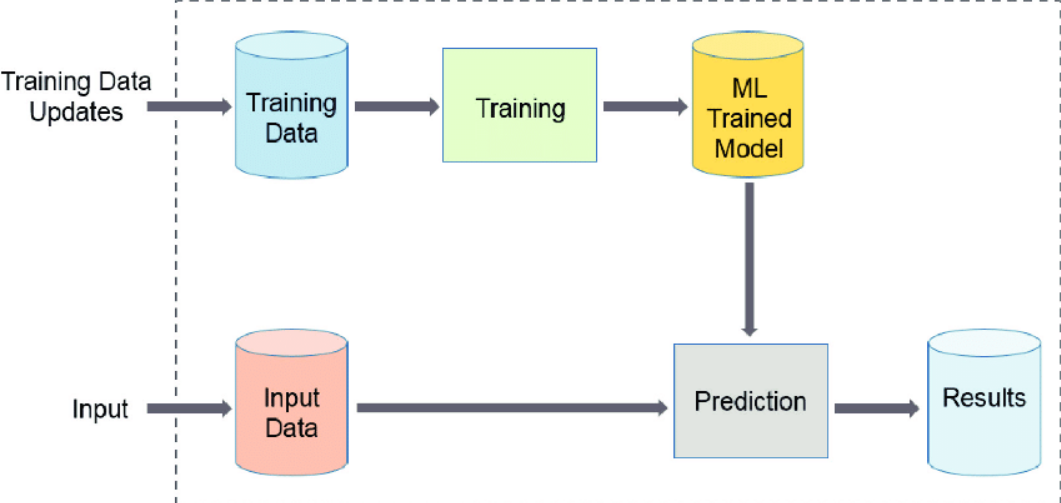Source: A-generic-machine-learning-workflow.png (850×407) (researchgate.net)

Let us consider a simple dataset in order to understand how ML algorithms work.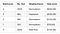Source: Machine Learning is Fun!. The world’s easiest introduction to… | by Adam Geitgey | Medium

Consider this input dataset also referred to as the ‘training set’. The different features such as: bedrooms, Sq. feet and Neighbourhood can be referred to as ‘x1’, ‘x2’ and ‘x3’. It must be understood that ‘x1’, ‘x2’ and ‘x3’ together constitute ‘x’ which is the input.

Here, we specify the feature ‘Sale price’ as ‘y’. This is an example of a Supervised Machine Learning Algorithm as we’re telling the algorithm which feature has to be treated as the output ‘y’.

After being fed with data, the model then implements an algorithm to determine a relationship between ‘x’ and ‘y’. Here, the algorithm is training the ML model to ‘think’ like a human brain.

After being trained, we can use this model for predicting outcomes of certain data.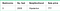Cost Function | Coursera

Now let’s say we wish to buy a 3-bedroom house that has an area of 2000 sq. feet and is located in Hipsterton but we don’t know its price. While we don’t have its price, what we do have is an ML model which can do the work of predicting its price. So, we feed these values as input to the ML model and it returns the predicted sale price of the house as ‘y’.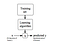Source: Model Representation | Coursera

## What Does an Algorithm Mean?

One might wonder what exactly does the term ‘algorithm’ mean here, in simple terms, ‘algorithm’ refers to the method the model uses to predict the output. For example, let’s consider a scatter plot for a simple dataset where x is the input, and y is the output: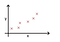Cost Function | Coursera

Now, let us consider an ML model which uses an algorithm known as ‘Linear Regression’. So, an ML model that implements such an algorithm will plot a straight line to ‘fit’ the data points and model a relationship between x and y.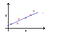Cost Function | Coursera

After fitting the data, the model is now trained and can be used for predicting outcomes. So, let us input another value of x.

Let this value of ‘x’ be given by the sky-blue spot along the x-axis. What the model will do is, it will fit ‘x’ onto the line as indicate by the sky-blue cross and determine the corresponding y-value on the y-axis. It will then return ‘y’ as output.

So, this was the working of a Supervised Machine Learning model that implements Linear Regression Algorithm. Similar to Linear Regression, we have another algorithm called Polynomial Regression which can be used when a straight plot fails to fit the data accurately.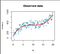polynomial regression graph — Bing images

## Why Do We Need Machine Learning ?

What makes Machine Learning so interesting are its real-life applications. Tesla uses ML to develop self-driving cars wherein the image of the road ahead acts as ‘x’ and rotation of the steering wheel along with acceleration/deceleration acts as ‘y’.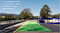machine learning in a self driving car — Bing images

Mail uses ML to classify our mail as spam/not spam using an Unsupervised Machine Learning Algorithm, wherein the ML model determines the relationships between spam mails on the basis of usage of words like ‘discount’, ‘sale’, ‘buy now’ etc. This way, it determines if a mail is spam or not spam without being explicitly told which is spam.

Netflix implements ML to suggest movies to us. Microsoft Edge uses ML to sort our news article on the basis of our interests.

While we are a long way away from developing ML models that can think like an actual human brain, we have achieved multiple breakthroughs in the field of Artificial Intelligence, while ML maybe a term that is not as common as football or cricket, its applications are undoubtedly widespread and large in number and cannot be ignored.

It is a fact that ‘change is the only constant’, as an engineer, I would love to rephrase it to ‘innovation is the only constant’ and innovation is indeed the solution to developing amazing ML models that can achieve great technological breakthroughs.